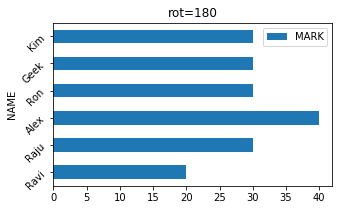# Pandas DataFrame Plot barh graph

Pandas plot

Python Pandas Plot horizontal or vertical Bar graph by using DataFrame with options & save as image

Pandas.DataFrame.plot to get bar graphs using data

Let us create a DataFrame with name of the students and their marks.``````import pandas as pd
my_dict={
'NAME':['Ravi','Raju','Alex',
'Ron','Geek','Kim'],
'MARK':[20,30,40,30,30,30]
}
df = pd.DataFrame(data=my_dict)
df.plot.barh(title="rot=180",x='NAME',
y='MARK',figsize=(5,3),rot=45)``````

## Bar Graph with options

There are several options we can add to above bar graph and they are same as plot.bar. So read the options of bar graph.

Subscribe to our YouTube Channel here

## Subscribe

* indicates required
Subscribe to plus2netplus2net.com